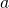# Random Questions from 2017 Prelims #3Here is another question that is from CJC H2 Mathematics 9758 Prelim Paper 1. Its a question on differentiation. I think it is simple enough and tests student on their thinking comprehension skills. This is question 6.

A straight line passes through the point with coordinates (4, 3) cuts the positive x-axis at point P and positive y-axis at point Q. It is given that, whereand O is the origin.

(i) Show that equation of line PQ is given by.

(ii) By finding an expression for, show that asvaries, the stationary value ofis, whereandare constants to be determined.

Not readable? Change text.